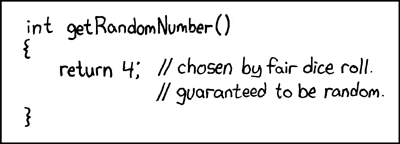## biased sample!

Posted in Statistics with tags , , , , , , , , , , , on May 21, 2019 by xi'anA chance occurrence led me to this thread on R-devel about R sample function generating a bias by taking the integer part of the continuous uniform generator… And then to the note by Kellie Ottoboni and Philip Stark analysing the reason, namely the fact that R uniform [0,1) pseudo-random generator is not perfectly continuously uniform but discrete, by the nature of numbers on a computer. Knuth (1997) showed that in this case the range of probabilities is larger than (1,1), the largest range being (1,1.03). As noted in the note, exploiting directly the pseudo-random bits of the pseudo-random generator. Shocking, isn’t it!  A fast and bias-free alternative suggested by Lemire is available as `dqsample::sample`

As an update of June 2019, sample is now fixed.

## complexity of the von Neumann algorithm

Posted in Statistics with tags , , , , , , , , , on April 3, 2017 by xi'an“Without the possibility of computing infimum and supremum of the density f over compact subintervals of the domain of f, sampling absolutely continuous distribution using the rejection method seems to be impossible in total generality.”

The von Neumann algorithm is another name for the rejection method introduced by von Neumann circa 1951. It was thus most exciting to spot a paper by Luc Devroye and Claude Gravel appearing in the latest Statistics and Computing. Assessing the method in terms of random bits and precision. Specifically, assuming that the only available random generator is one of random bits, which necessarily leads to an approximation when the target is a continuous density. The authors first propose a bisection algorithm for distributions defined on a compact interval, which compares random bits with recursive bisections of the unit interval and stops when the interval is small enough.In higher dimension, for densities f over the unit hypercube, they recall that the original algorithm consisted in simulating uniforms x and u over the hypercube and [0,1], using the uniform as the proposal distribution and comparing the density at x, f(x), with the rescaled uniform. When using only random bits, the proposed method is based on a quadtree that subdivides the unit hypercube into smaller and smaller hypercubes until the selected hypercube is entirely above or below the density. And is small enough for the desired precision. This obviously requires for the computation of the upper and lower bound of the density over the hypercubes to be feasible, with Devroye and Gravel considering that this is a necessary property as shown by the above quote. Densities with non-compact support can be re-expressed as densities on the unit hypercube thanks to the cdf transform. (Actually, this is equivalent to the general accept-reject algorithm, based on the associated proposal.)

“With the oracles introduced in our modification of von Neumann’s method, we believe that it is impossible to design a rejection algorithm for densities that are not Riemann-integrable, so the question of the design of a universally valid rejection algorithm under the random bit model remains open.”

In conclusion, I enjoyed very much reading this paper, especially the reflection it proposes on the connection between Riemann integrability and rejection algorithms. (Actually, I cannot think straight away of a simulation algorithm that would handle non-Riemann-integrable densities, apart from nested sampling. Or of significant non-Riemann-integrable densities.)